# PSEB 7th Class Maths Solutions Chapter 10 Practical Geometry Ex 10.4

Punjab State Board PSEB 7th Class Maths Book Solutions Chapter 10 Practical Geometry Ex 10.4 Textbook Exercise Questions and Answers.

## PSEB Solutions for Class 7 Maths Chapter 10 Practical Geometry Ex 10.4

1. Construct ΔABC, given AB = 6 cm, ∠A = 30° and ∠B = 75°.
Solution:
Given. One side of ΔABC as AB = 6 cm, m∠A = 30° and m∠B = 75°.
To construct: A triangle with one side and these two angles.
Steps of Construction :
Step 1. We first draw a rough sketch of ΔABC and indicate the measures of side and two angles.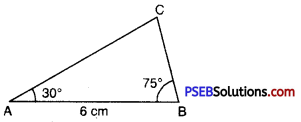Step 2. Draw a ray AB of length 6 cm.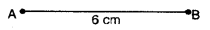Step 3. At A; draw a ray AX making an angle 30° with AB.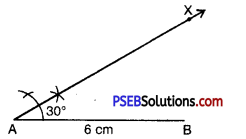Step 4. With the help of compass. At B; draw a ray BY making an angle of 75° with AB.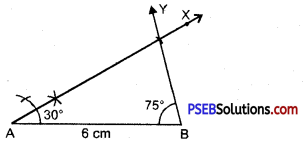Step 5. Both rays AX and BY intersect, at a point. So the point of intersection of the two rays is C Then. ΔABC is now obtained.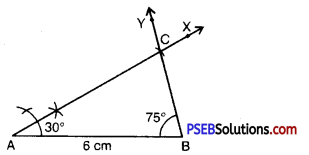2. Construct an isosceles ΔABC such that AB = 5.3 cm and each base angle = 45°.
Solution:
Given : Isosceles ΔABC with AB = 5.3 cm each base angle = 45°.
To Construct: A triangle with one side and two base angles.
Steps of construction :
Step 1. Draw a rough sketch of ΔABC with given measures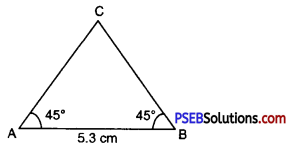Step 2. Draw a line segment AB = 5.3 cm.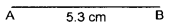Step 3. Taking A as centre with the help of compass. Draw a ray AX making an angle 45° with AB.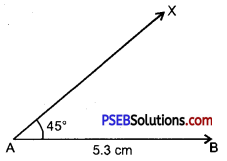Step 4. With the help of compass and taking B as a centre. Draw a ray BY making an angle 45° with the line segment AB.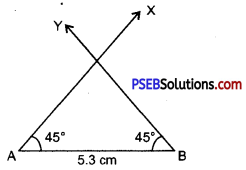Step 5. Rays AX and BY intersect, at a point say C, then ABC is the required triangle.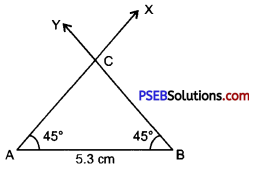3. Construct ΔXYZ if XY = 4 cm, ∠X = 45° and ∠Z = 60°.
[Hint : ∠Y = 180° – 45° – 60° = 75°]
Solution:
One side of ΔXYZ as
XY = 4 cm,
∠X = 45°
and ∠Z = 60°.
As we know that by angle-sum property of a triangle; sum of all three angles of a triangle is equal to 180°.
∴ ∠X + ∠Y + ∠Z = 180°
⇒ 45° + ∠Y + 60° = 180°
⇒ 105° + ∠Y = 180°
⇒ ∠Y = 75°.
Now it will be easy to construct triangle with side
XY = 4 cm,
∠X = 45°
and ∠Y = 75°.
Steps of Construction :
Step 1. We first draw a rough sketch of ΔXYZ and indicate the measure of side and two angles.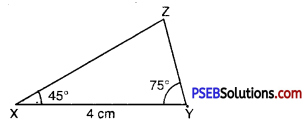Step 2. Draw a ray XY of length 4 cm.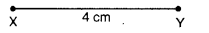Step 3. At X draw a ray XA making an angle of 45° with XY.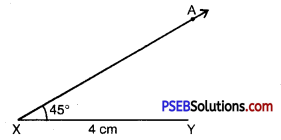Step 4. At Y; draw a ray YB making an angle of 75° with XY.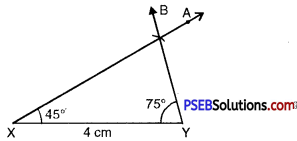Step 5. Z has to lie on both rays XA and YB. So, the point of intersection of two rays is Z.
ΔXYZ is now obtained.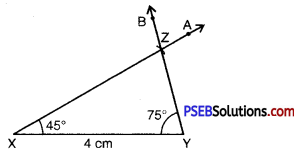4. Examine whether you can construct ΔPQR such that ∠P = 100°, ∠Q = 90° and PQ = 4.3 cm If not possible given reason.
Solution:
No, we cannot construct given ΔPQR.
Reason :
As we know that by angle sum property of a triangle; sum of all three angles a triangle is equal to 180°. But in given question sum of two angles;
m∠P + m∠Q
= 100° + 90°
= 190°
The sum of these two angles should be less than 180°. So triangle with given measures cannot be constructed as it violates the angle sum property of a triangle.

5. Question (i).
In which of the following cases a unique triangle can be drawn ?
(a) BC = 5 cm, ∠B = 90° and ∠C = 100°
(b) AB = 4 cm, BC = 7 cm and CA = 2 cm
(c) XY = 5 cm, ∠X = 45°, ∠Y = 60°
(d) An isosceles triangle with length of each equal side equal to 5 cm.